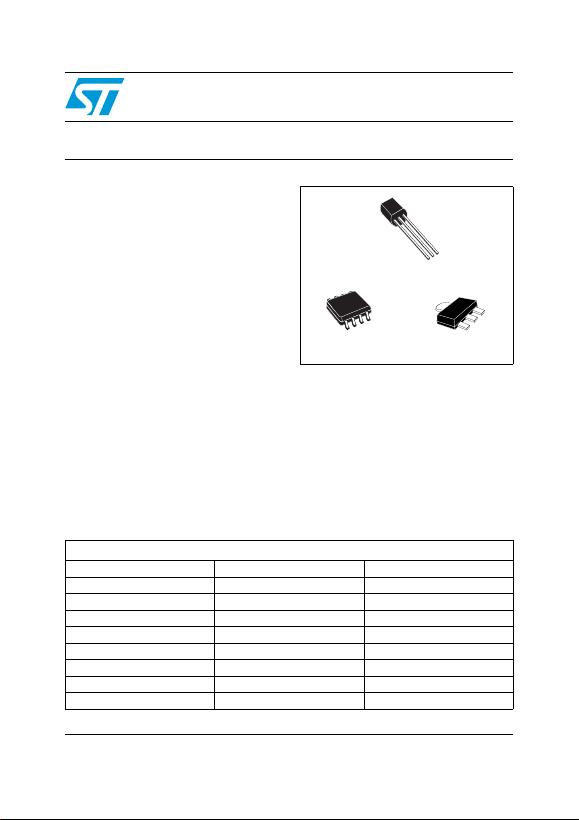This is information on a product in full production.
August 2012 Doc ID 2145 Rev 20 1/30
30
L78LxxAB,
L78LxxAC, L78LxxC
Positive voltage regulators
Datasheet — production data
Features
Output current up to 100 mA
Output voltages of 3.3; 5; 6; 8; 9; 10; 12; 15; 18;
24 V
Short-circuit protection
No external components are required
Available in either ± 4% (AC) or ± 8% (C)
selection
Description
The L78Lxx series of three-terminal positive
regulators employ internal current limiting and
thermal shutdown, making them essentially
indestructible. If adequate heat-sink is provided,
they can deliver up to 100 mA output current.
They are intended as fixed voltage regulators in a
wide range of applications including local or on-
card regulation for elimination of noise and
distribution problems associated with single-point
regulation. In addition, they can be used with
power pass elements to make high-current
voltage regulators. The L78Lxx series used as
Zener diode/resistor combination replacement,
offers an effective output impedance improvement
of typically two orders of magnitude, along with
lower quiescent current and lower noise.
SOT-89
TO-92
SO-8
Table 1. Device summary
Part numbers
L78L33C L78L08AC L78L15C
L78L33AC L78L08AB L78L15AC
L78L33AB L78L09C L78L15AB
L78L05C L78L09AC L78L18C
L78L05AC L78L09AB L78L18AC
L78L05AB L78L10AC L78L24C
L78L06AC L78L12C L78L24AC
L78L06AB L78L12AC L78L24AB
L78L08C L78L12AB
www.st.comContents L78LxxAB, L78LxxAC, L78LxxC
2/30 Doc ID 2145 Rev 20
Contents
1 Diagram . . . . . . . . . . . . . . . . . . . . . . . . . . . . . . . . . . . . . . . . . . . . . . . . . . . 3
2 Pin configuration . . . . . . . . . . . . . . . . . . . . . . . . . . . . . . . . . . . . . . . . . . . 4
3 Maximum ratings . . . . . . . . . . . . . . . . . . . . . . . . . . . . . . . . . . . . . . . . . . . . 5
4 Electrical characteristics . . . . . . . . . . . . . . . . . . . . . . . . . . . . . . . . . . . . . 6
5 Typical performance . . . . . . . . . . . . . . . . . . . . . . . . . . . . . . . . . . . . . . . . 16
6 Typical application . . . . . . . . . . . . . . . . . . . . . . . . . . . . . . . . . . . . . . . . . 18
7 Package mechanical data . . . . . . . . . . . . . . . . . . . . . . . . . . . . . . . . . . . . 20
8 Order codes . . . . . . . . . . . . . . . . . . . . . . . . . . . . . . . . . . . . . . . . . . . . . . . 28
9 Revision history . . . . . . . . . . . . . . . . . . . . . . . . . . . . . . . . . . . . . . . . . . . 29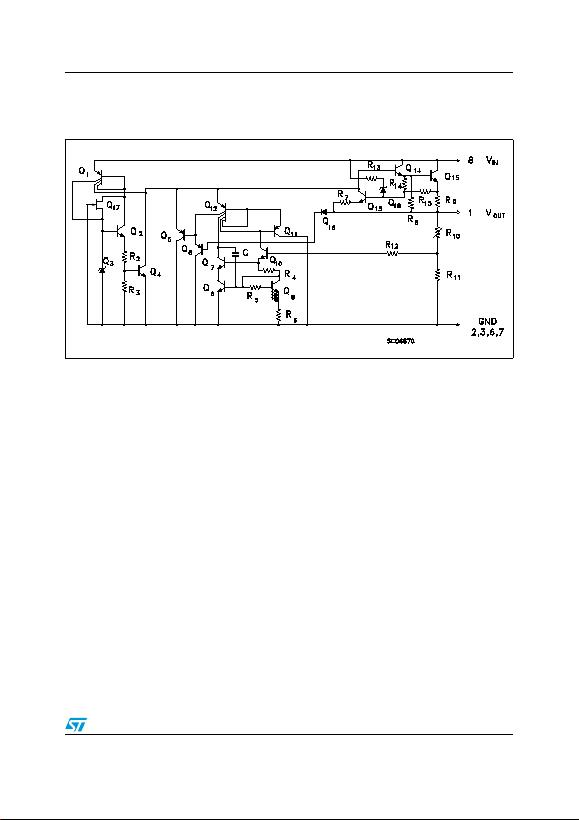L78LxxAB, L78LxxAC, L78LxxC Diagram
Doc ID 2145 Rev 20 3/30
1 Diagram
Figure 1. Schematic diagram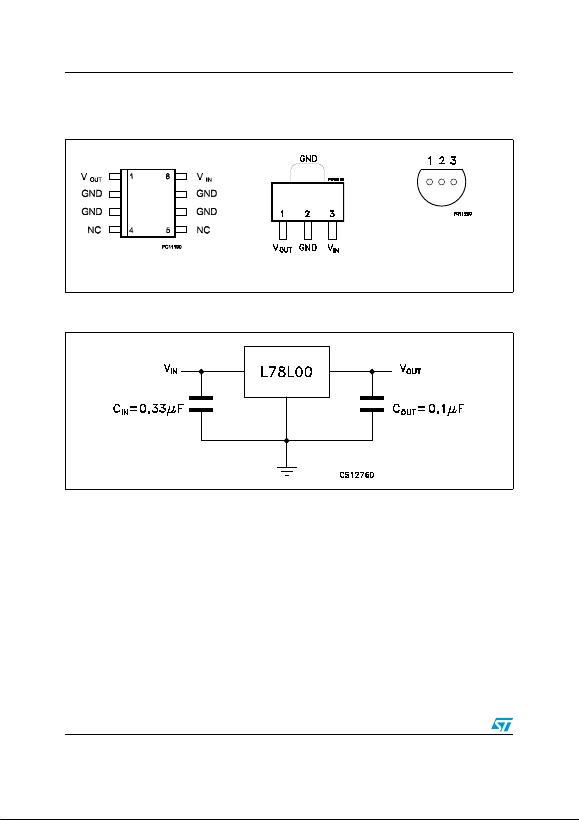Pin configuration L78LxxAB, L78LxxAC, L78LxxC
4/30 Doc ID 2145 Rev 20
2 Pin configuration
Figure 2. Pin connection (top view, bottom view for TO-92)
SOT-89
SO-8 TO-92
PIN 1 = VOUT
PIN 2 = GND
PIN 3 = VIN
Figure 3. Test circuits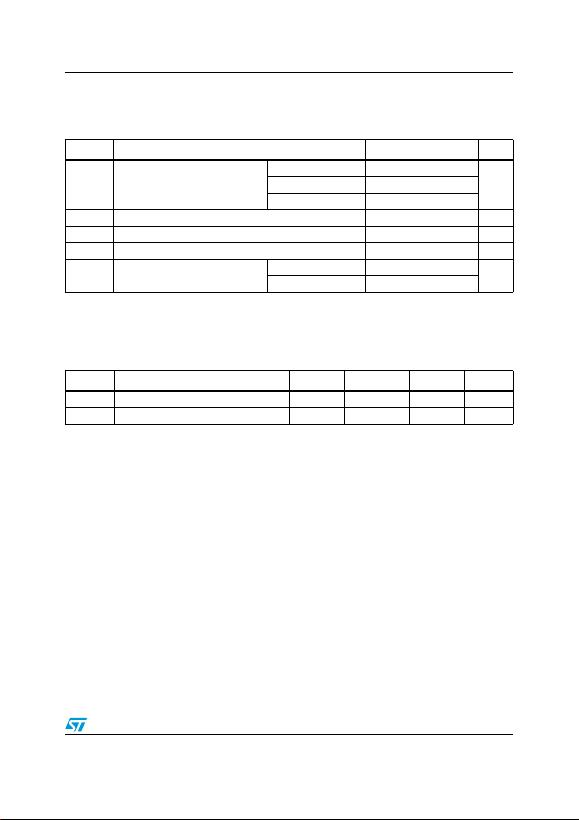L78LxxAB, L78LxxAC, L78LxxC Maximum ratings
Doc ID 2145 Rev 20 5/30
3 Maximum ratings
Table 2. Absolute maximum ratings
Symbol Parameter Value Unit
VIDC Input voltage
VO= 3.3 to 9 V 30
VVO= 12 to 15 V 35
VO= 18 to 24 V 40
IOOutput current 100 mA
PDPower dissipation Internally limited (1)
1. Our SO-8 package used for voltage regulators is modified internally to have pins 2, 3, 6 and 7 electrically communed to the
die attach flag. This particular frame decreases the total thermal resistance of the package and increases its ability to
dissipate power when an appropriate area of copper on the printed circuit board is available for heat-sinking. The external
dimensions are the same as for the standard SO-8.
mW
TSTG Storage temperature range -65 to 150 °C
TOP Operating junction temperature range for L78L00AC 0 to 125 °C
for L78L00AB -40 to 125
Table 3. Thermal data
Symbol Parameter SO-8 TO-92 SOT-89 Unit
RthJC Thermal resistance junction-case. (max) 20 15 °C/W
RthJA Thermal resistance junction-ambient. (max) 55 (1) 200 55 (1) °C/W
1. Considering 6 cm² of copper Board heat-sink.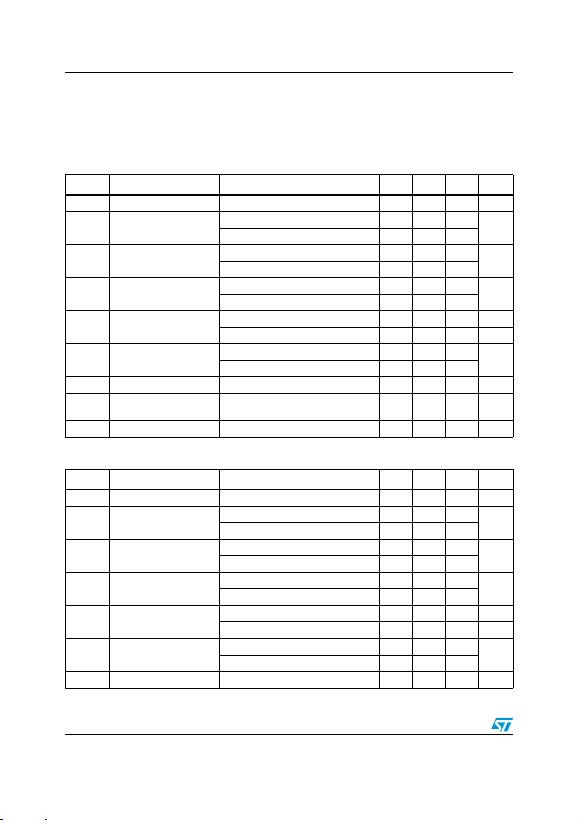Electrical characteristics L78LxxAB, L78LxxAC, L78LxxC
6/30 Doc ID 2145 Rev 20
4 Electrical characteristics
Refer to the test circuits, TJ = 0 to 125 °C, IO = 40 mA, CI = 0.33 µF, CO = 0.1 µF unless
otherwise specified.
Table 4. Electrical characteristics of L78L33C (VI = 8.3 V)
Symbol Parameter Test conditions Min. Typ. Max. Unit
VOOutput voltage TJ = 25 °C 3.036 3.3 3.564 V
VOOutput voltage IO = 1 to 40 mA, VI = 5.3 to 20 V 2.97 3.63 V
IO = 1 to 70 mA, VI = 8.3 V 2.97 3.63
ΔVOLine regulation VI = 5.3 to 20 V, TJ = 25 °C 150 mV
VI = 6.3 to 20 V, TJ = 25 °C 100
ΔVOLoad regulation IO = 1 to 100 mA, TJ = 25 °C 60 mV
IO = 1 to 40 mA, TJ = 25 °C 30
IdQuiescent current TJ = 25 °C 6 mA
TJ = 125 °C 5.5 mA
ΔIdQuiescent current change IO = 1 to 40 mA 0.2 mA
VI = 6.3 to 20 V 1.5
eN Output noise voltage B =10 Hz to 100 kHz, TJ = 25 °C 40 µV
SVR Supply voltage rejection VI = 6.3 to 16.3 V, f = 120 Hz
IO = 40 mA, TJ = 25 °C 41 49 dB
VdDropout voltage 1.7 V
Table 5. Electrical characteristics of L78L05C (VI = 10 V)
Symbol Parameter Test conditions Min. Typ. Max. Unit
VOOutput voltage TJ = 25 °C 4.6 5 5.4 V
VOOutput voltage IO = 1 to 40 mA, VI = 7 to 20 V 4.5 5.5 V
IO = 1 to 70 mA, VI = 10 V 4.5 5.5
ΔVOLine regulation VI = 8.5 to 20 V, TJ = 25 °C 200 mV
VI = 9 to 20 V, TJ = 25 °C 150
ΔVOLoad regulation IO = 1 to 100 mA, TJ = 25 °C 60 mV
IO = 1 to 40 mA, TJ = 25 °C 30
IdQuiescent current TJ = 25 °C 6 mA
TJ = 125 °C 5.5 mA
ΔIdQuiescent current change IO = 1 to 40 mA 0.2 mA
VI = 8 to 20 V 1.5
eN Output noise voltage B =10 Hz to 100 kHz, TJ = 25 °C 40 µV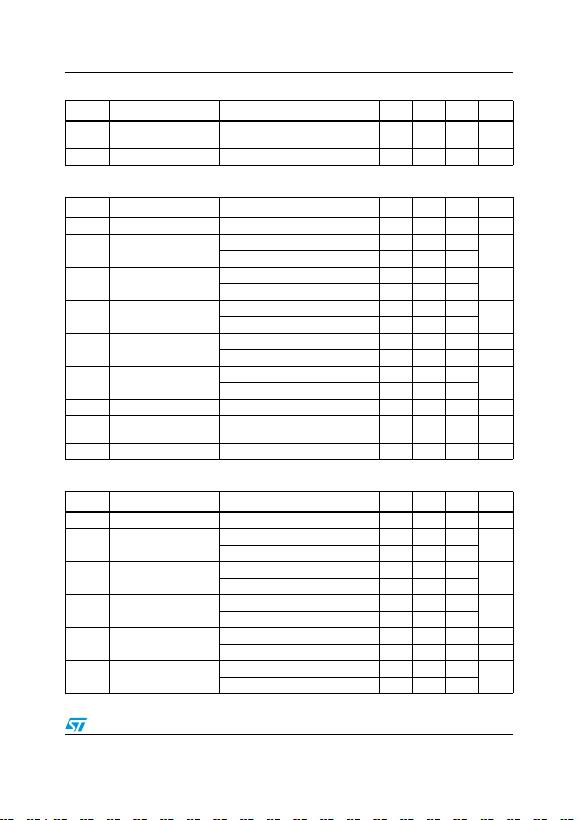L78LxxAB, L78LxxAC, L78LxxC Electrical characteristics
Doc ID 2145 Rev 20 7/30
SVR Supply voltage rejection VI = 9 to 20 V, f = 120 Hz
IO = 40 mA, TJ = 25 °C 40 49 dB
VdDropout voltage 1.7 V
Table 5. Electrical characteristics of L78L05C (VI = 10 V) (continued)
Symbol Parameter Test conditions Min. Typ. Max. Unit
Table 6. Electrical characteristics of L78L08C (VI = 14 V)
Symbol Parameter Test conditions Min. Typ. Max. Unit
VOOutput voltage TJ = 25 °C 7.36 8 8.64 V
VOOutput voltage IO = 1 to 40 mA, VI = 8.5 to 20 V 7.2 8.8 V
IO = 1 to 70 mA, VI = 12 V 7.2 8.8
ΔVOLine regulation VI = 8.5 to 20 V, TJ = 25 °C 200 mV
VI = 9 to 20 V, TJ = 25 °C 150
ΔVOLoad regulation IO = 1 to 100 mA, TJ = 25 °C 80 mV
IO = 1 to 40 mA, TJ = 25 °C 40
IdQuiescent current TJ = 25 °C 6 mA
TJ = 125 °C 5.5 mA
ΔIdQuiescent current change IO = 1 to 40 mA 0.2 mA
VI = 8 to 20 V 1.5
eN Output noise voltage B =10 Hz to 100 kHz, TJ = 25 °C 60 µV
SVR Supply voltage rejection VI = 9 to 20 V, f = 120 Hz
IO = 40 mA, TJ = 25 °C 36 45 dB
VdDropout voltage 1.7 V
Table 7. Electrical characteristics of L78L09C (VI = 15 V)
Symbol Parameter Test conditions Min. Typ. Max. Unit
VOOutput voltage TJ = 25 °C 8.28 9 9.72 V
VOOutput voltage IO = 1 to 40 mA, VI = 11.5 to 23 V 8.1 9.9 V
IO = 1 to 70 mA, VI = 15 V 8.1 9.9
ΔVOLine regulation VI = 11.5 to 23 V, TJ = 25 °C 250 mV
VI = 12 to 23 V, TJ = 25 °C 200
ΔVOLoad regulation IO = 1 to 100 mA, TJ = 25 °C 80 mV
IO = 1 to 40 mA, TJ = 25 °C 40
IdQuiescent current TJ = 25 °C 6 mA
TJ = 125 °C 5.5 mA
ΔIdQuiescent current change IO = 1 to 40 mA 0.2 mA
VI = 12 to 23 V 1.5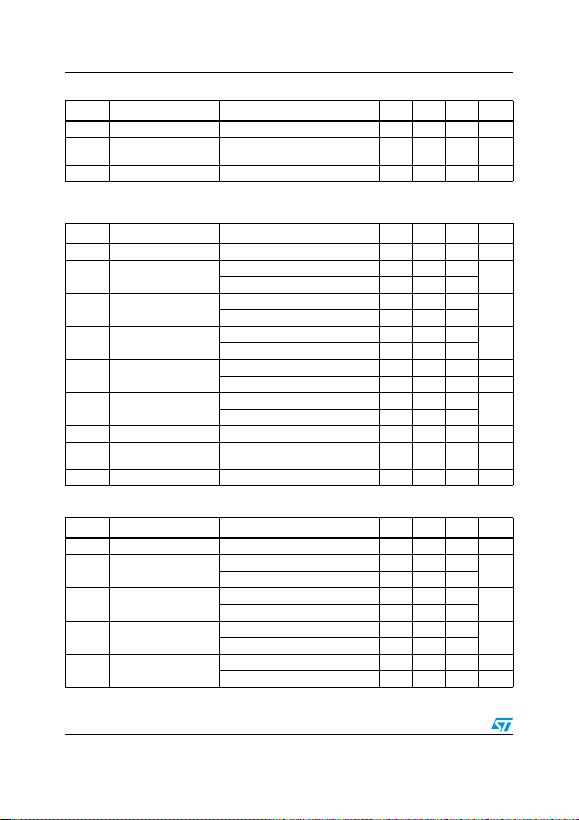Electrical characteristics L78LxxAB, L78LxxAC, L78LxxC
8/30 Doc ID 2145 Rev 20
eN Output noise voltage B =10 Hz to 100 kHz, TJ = 25 °C 70 µV
SVR Supply voltage rejection VI = 12 to 23 V, f = 120 Hz
IO = 40 mA, TJ = 25 °C 36 44 dB
VdDropout voltage 1.7 V
Table 7. Electrical characteristics of L78L09C (VI = 15 V) (continued)
Symbol Parameter Test conditions Min. Typ. Max. Unit
Table 8. Electrical characteristics of L78L10C (VI = 16 V)
Symbol Parameter Test conditions Min. Typ. Max. Unit
VOOutput voltage TJ = 25 °C 9.2 10 10.8 V
VOOutput voltage IO = 1 to 40 mA, VI = 12.5 to 23 V 9 11 V
IO = 1 to 70 mA, VI = 16 V 9 11
ΔVOLine regulation VI = 12.5 to 23 V, TJ = 25 °C 230 mV
VI = 13 to 23 V, TJ = 25 °C 170
ΔVOLoad regulation IO = 1 to 100 mA, TJ = 25 °C 80 mV
IO = 1 to 40 mA, TJ = 25 °C 40
IdQuiescent current TJ = 25 °C 6 mA
TJ = 125 °C 5.5 mA
ΔIdQuiescent current change IO = 1 to 40 mA 0.1 mA
VI = 13 to 23 V 1.5
eN Output noise voltage B =10Hz to 100kHz, TJ = 25 °C 60 µV
SVR Supply voltage rejection VI = 14 to 23 V, f = 120Hz
IO = 40 mA, TJ = 25 °C 37 45 dB
VdDropout voltage 1.7 V
Table 9. Electrical characteristics of L78L12C (VI = 19 V)
Symbol Parameter Test conditions Min. Typ. Max. Unit
VOOutput voltage TJ = 25 °C 11.1 12 12.9 V
VOOutput voltage IO = 1 to 40 mA, VI = 14.5 to 27 V 10.8 13.2 V
IO = 1 to 70 mA, VI = 19 V 10.8 13.2
ΔVOLine regulation VI = 14.5 to 27 V, TJ = 25 °C 250 mV
VI = 16 to 27 V, TJ = 25 °C 200
ΔVOLoad regulation IO = 1 to 100 mA, TJ = 25 °C 100 mV
IO = 1 to 40 mA, TJ = 25 °C 50
IdQuiescent current TJ = 25 °C 6.5 mA
TJ = 125 °C 6 mA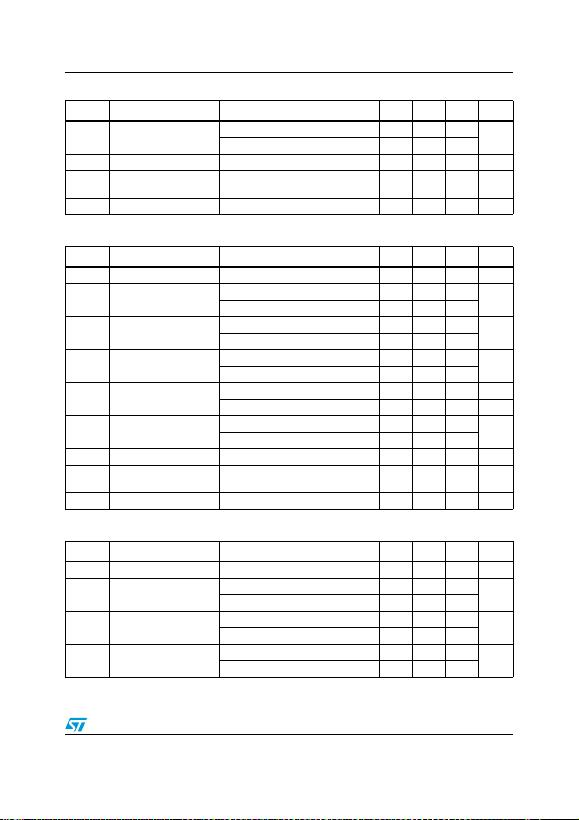L78LxxAB, L78LxxAC, L78LxxC Electrical characteristics
Doc ID 2145 Rev 20 9/30
ΔIdQuiescent current change IO = 1 to 40 mA 0.2 mA
VI = 16 to 27 V 1.5
eN Output noise voltage B =10 Hz to 100 kHz, TJ = 25 °C 80 µV
SVR Supply voltage rejection VI = 15 to 25 V, f = 120 Hz
IO = 40 mA, TJ = 25 °C 36 42 dB
VdDropout voltage 1.7 V
Table 9. Electrical characteristics of L78L12C (VI = 19 V) (continued)
Symbol Parameter Test conditions Min. Typ. Max. Unit
Table 10. Electrical characteristics of L78L15C (VI = 23 V)
Symbol Parameter Test conditions Min. Typ. Max. Unit
VOOutput voltage TJ = 25 °C 13.8 15 16.2 V
VOOutput voltage IO = 1 to 40 mA, VI = 17.5 to 30 V 13.5 16.5 V
IO = 1 to 70 mA, VI = 23 V 13.5 16.5
ΔVOLine regulation VI = 17.5 to 30 V, TJ = 25 °C 300 mV
VI = 20 to 30 V, TJ = 25 °C 250
ΔVOLoad regulation IO = 1 to 100 mA, TJ = 25 °C 150 mV
IO = 1 to 40 mA, TJ = 25 °C 75
IdQuiescent current TJ = 25 °C 6.5 mA
TJ = 125 °C 6 mA
ΔIdQuiescent current change IO = 1 to 40 mA 0.2 mA
VI = 20 to 30 V 1.5
eN Output noise voltage B =10 Hz to 100 kHz, TJ = 25 °C 90 µV
SVR Supply voltage rejection VI = 18.5 to 28.5 V, f = 120 Hz
IO = 40 mA, TJ = 25 °C 33 39 dB
VdDropout voltage 1.7 V
Table 11. Electrical characteristics of L78L18C (VI = 27 V)
Symbol Parameter Test conditions Min. Typ. Max. Unit
VOOutput voltage TJ = 25 °C 16.6 18 19.4 V
VOOutput voltage IO = 1 to 40 mA, VI = 22 to 33 V 16.2 19.8 V
IO = 1 to 70 mA, VI = 27 V 16.2 19.8
ΔVOLine regulation VI = 22 to 33 V, TJ = 25 °C 320 mV
VI = 22 to 33 V, TJ = 25 °C 270
ΔVOLoad regulation IO = 1 to 100 mA, TJ = 25 °C 170 mV
IO = 1 to 40 mA, TJ = 25 °C 85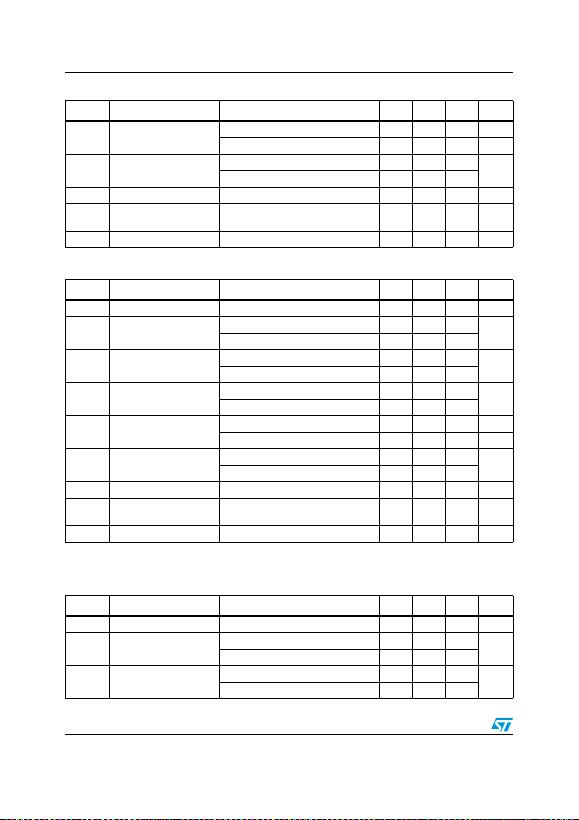Electrical characteristics L78LxxAB, L78LxxAC, L78LxxC
10/30 Doc ID 2145 Rev 20
TJ = 0 to 125 °C for L78L33AC, TJ = -40 to 125 °C for L78L33AB.
IdQuiescent current TJ = 25 °C 6.5 mA
TJ = 125 °C 6 mA
ΔIdQuiescent current change IO = 1 to 40 mA 0.2 mA
VI = 23 to 33 V 1.5
eN Output noise voltage B =10 Hz to 100 kHz, TJ = 25 °C 120 µV
SVR Supply voltage rejection VI = 23 to 33 V, f = 120 Hz
IO = 40 mA, TJ = 25 °C 32 38 dB
VdDropout voltage 1.7 V
Table 11. Electrical characteristics of L78L18C (VI = 27 V) (continued)
Symbol Parameter Test conditions Min. Typ. Max. Unit
Table 12. Electrical characteristics of L78L24C (VI = 33 V)
Symbol Parameter Test conditions Min. Typ. Max. Unit
VOOutput voltage TJ = 25 °C 22.1 24 25.9 V
VOOutput voltage IO = 1 to 40 mA, VI = 27 to 38 V 21.6 26.4 V
IO = 1 to 70 mA, VI = 33 V 21.6 26.4
ΔVOLine regulation VI = 27 to 38 V, TJ = 25 °C 350 mV
VI = 28 to 38 V, TJ = 25 °C 300
ΔVOLoad regulation IO = 1 to 100 mA, TJ = 25 °C 200 mV
IO = 1 to 40 mA, TJ = 25 °C 100
IdQuiescent current TJ = 25 °C 6.5 mA
TJ = 125 °C 6 mA
ΔIdQuiescent current change IO = 1 to 40 mA 0.2 mA
VI = 28 to 38 V 1.5
eN Output noise voltage B =10 Hz to 100 kHz, TJ = 25 °C 200 µV
SVR Supply voltage rejection VI = 29 to 35 V, f = 120 Hz
IO = 40 mA, TJ = 25 °C 30 37 dB
VdDropout voltage 1.7 V
Table 13. Electrical characteristics of L78L33AB and L78L33AC (VI = 8.3 V)
Symbol Parameter Test conditions Min. Typ. Max. Unit
VOOutput voltage TJ = 25 °C 3.168 3.3 3.432 V
VOOutput voltage IO = 1 to 40 mA, VI = 5.3 to 20 V 3.135 3.465 V
IO = 1 to 70 mA, VI = 8.3 V 3.135 3.465
ΔVOLine regulation VI = 5.3 to 20 V, TJ = 25 °C 150 mV
VI = 6.3 to 20 V, TJ = 25 °C 100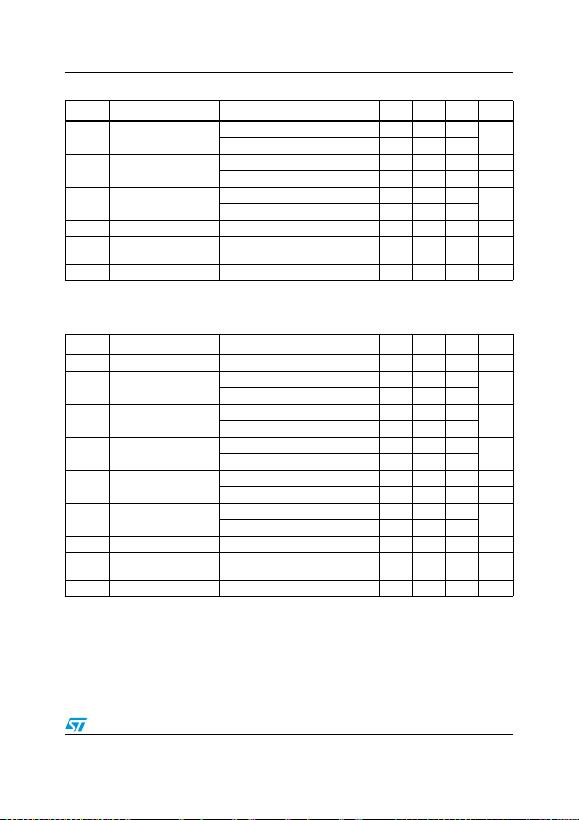L78LxxAB, L78LxxAC, L78LxxC Electrical characteristics
Doc ID 2145 Rev 20 11/30
TJ = 0 to 125 °C for L78L05AC, TJ = -40 to 125 °C for L78L05AB.
TJ = 0 to 125 °C for L78L06AC, TJ = -40 to 125 °C for L78L06AB.
ΔVOLoad regulation IO = 1 to 100 mA, TJ = 25 °C 60 mV
IO = 1 to 40 mA, TJ = 25 °C 30
IdQuiescent current TJ = 25 °C 6 mA
TJ = 125 °C 5.5 mA
ΔIdQuiescent current change IO = 1 to 40 mA 0.1 mA
VI = 6.3 to 20 V 1.5
eN Output noise voltage B =10 Hz to 100 kHz, TJ = 25 °C 40 µV
SVR Supply voltage rejection VI = 6.3 to 16.3 V, f = 120 Hz
IO = 40 mA, TJ = 25 °C 41 49 dB
VdDropout voltage 1.7 V
Table 13. Electrical characteristics of L78L33AB and L78L33AC (VI = 8.3 V) (continued)
Symbol Parameter Test conditions Min. Typ. Max. Unit
Table 14. Electrical characteristics of L78L05AB and L78L05AC (VI = 10 V)
Symbol Parameter Test conditions Min. Typ. Max. Unit
VOOutput voltage TJ = 25 °C 4.8 5 5.2 V
VOOutput voltage IO = 1 to 40 mA, VI = 7 to 20 V 4.75 5.25 V
IO = 1 to 70 mA, VI = 10 V 4.75 5.25
ΔVOLine regulation VI = 7 to 20 V, TJ = 25 °C 150 mV
VI = 8 to 20 V, TJ = 25 °C 100
ΔVOLoad regulation IO = 1 to 100 mA, TJ = 25 °C 60 mV
IO = 1 to 40 mA, TJ = 25 °C 30
IdQuiescent current TJ = 25 °C 6 mA
TJ = 125 °C 5.5 mA
ΔIdQuiescent current change IO = 1 to 40 mA 0.1 mA
VI = 8 to 20 V 1.5
eN Output noise voltage B =10 Hz to 100 kHz, TJ = 25 °C 40 µV
SVR Supply voltage rejection VI = 8 to 18 V, f = 120 Hz
IO = 40 mA, TJ = 25 °C 41 49 dB
VdDropout voltage 1.7 V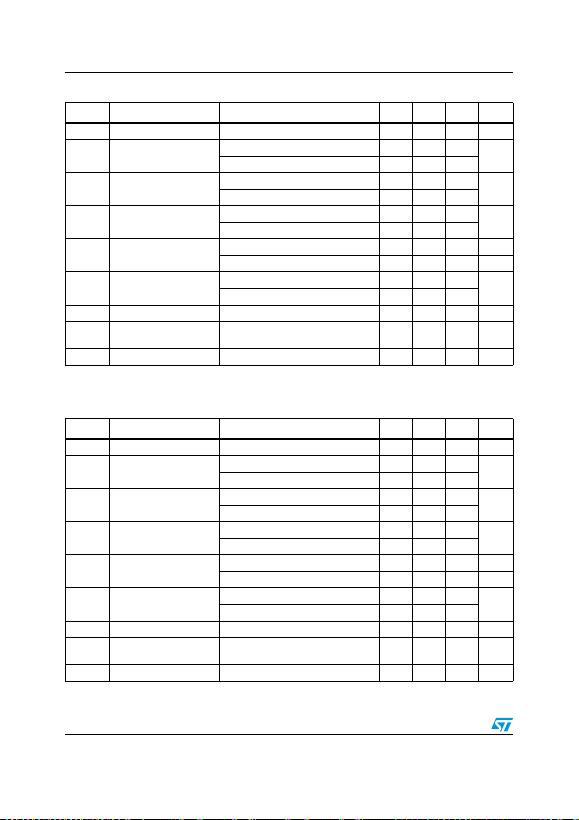Electrical characteristics L78LxxAB, L78LxxAC, L78LxxC
12/30 Doc ID 2145 Rev 20
TJ = 0 to 125 °C for L78L08AC, TJ = -40 to 125 °C for L78L08AB.
Table 15. Electrical characteristics of L78L06AB and L78L06AC (VI = 12 V)
Symbol Parameter Test conditions Min. Typ. Max. Unit
VOOutput voltage TJ = 25 °C 5.76 6 6.24 V
VOOutput voltage IO = 1 to 40 mA, VI = 8.5 to 20 V 5.7 6.3 V
IO = 1 to 70 mA, VI = 12 V 5.7 6.3
ΔVOLine regulation VI = 8.5 to 20 V, TJ = 25 °C 150 mV
VI = 9 to 20 V, TJ = 25 °C 100
ΔVOLoad regulation IO = 1 to 100 mA, TJ = 25 °C 60 mV
IO = 1 to 40 mA, TJ = 25 °C 30
IdQuiescent current TJ = 25 °C 6 mA
TJ = 125 °C 5.5 mA
ΔIdQuiescent current change IO = 1 to 40 mA 0.1 mA
VI = 9 to 20 V 1.5
eN Output noise voltage B =10 Hz to 100 kHz, TJ = 25 °C 50 µV
SVR Supply voltage rejection VI = 9 to 20 V, f = 120 Hz
IO = 40 mA, TJ = 25 °C 39 46 dB
VdDropout voltage 1.7 V
Table 16. Electrical characteristics of L78L08AB and L78L08AC (VI = 14 V)
Symbol Parameter Test conditions Min. Typ. Max. Unit
VOOutput voltage TJ = 25 °C 7.68 8 8.32 V
VOOutput voltage IO = 1 to 40 mA, VI = 10.5 to 23 V 7.6 8.4 V
IO = 1 to 70 mA, VI = 14 V 7.6 8.4
ΔVOLine regulation VI = 10.5 to 23 V, TJ = 25 °C 175 mV
VI = 11 to 23 V, TJ = 25 °C 125
ΔVOLoad regulation IO = 1 to 100 mA, TJ = 25 °C 80 mV
IO = 1 to 40 mA, TJ = 25 °C 40
IdQuiescent current TJ = 25 °C 6 mA
TJ = 125 °C 5.5 mA
ΔIdQuiescent current change IO = 1 to 40 mA 0.1 mA
VI = 11 to 23 V 1.5
eN Output noise voltage B =10 Hz to 100 kHz, TJ = 25 °C 60 µV
SVR Supply voltage rejection VI = 12 to 23 V, f = 120 Hz
IO = 40 mA, TJ = 25 °C 37 45 dB
VdDropout voltage 1.7 V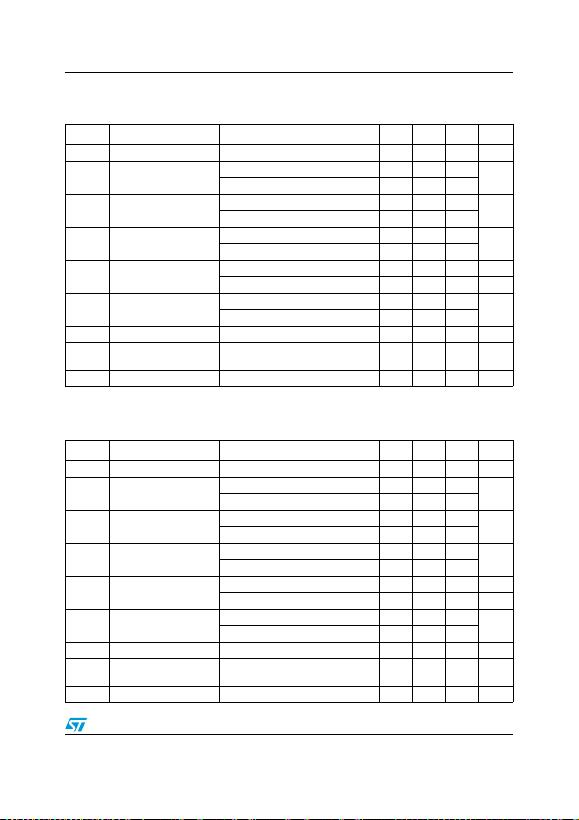L78LxxAB, L78LxxAC, L78LxxC Electrical characteristics
Doc ID 2145 Rev 20 13/30
TJ = 0 to 125 °C for L78L09AC, TJ = -40 to 125 °C for L78L09AB.
TJ = 0 to 125 °C for L78L10AC, TJ = -40 to 125 °C for L78L10AB.
Table 17. Electrical characteristics of L78L09AB and L78L09AC (VI = 15 V)
Symbol Parameter Test conditions Min. Typ. Max. Unit
VOOutput voltage TJ = 25 °C 8.64 9 9.36 V
VOOutput voltage IO = 1 to 40 mA, VI = 11.5 to 23 V 8.55 9.45 V
IO = 1 to 70 mA, VI = 15 V 8.55 9.45
ΔVOLine regulation VI = 11.5 to 23 V, TJ = 25 °C 225 mV
VI = 12 to 23 V, TJ = 25 °C 150
ΔVOLoad regulation IO = 1 to 100 mA, TJ = 25 °C 80 mV
IO = 1 to 40 mA, TJ = 25 °C 40
IdQuiescent current TJ = 25 °C 6 mA
TJ = 125 °C 5.5 mA
ΔIdQuiescent current change IO = 1 to 40 mA 0.1 mA
VI = 12 to 23 V 1.5
eN Output noise voltage B =10 Hz to 100 kHz, TJ = 25 °C 70 µV
SVR Supply voltage rejection VI = 12 to 23 V, f = 120 Hz
IO = 40 mA, TJ = 25 °C 37 44 dB
VdDropout voltage 1.7 V
Table 18. Electrical characteristics of L78L10AC (VI = 16 V)
Symbol Parameter Test conditions Min. Typ. Max. Unit
VOOutput voltage TJ = 25 °C 9.6 10 10.4 V
VOOutput voltage IO = 1 to 40 mA, VI = 12.5 to 23 V 9.5 10.5 V
IO = 1 to 70 mA, VI = 16 V 9.5 10.5
ΔVOLine regulation VI = 12.5 to 23 V, TJ = 25 °C 230 mV
VI = 13 to 23 V, TJ = 25 °C 170
ΔVOLoad regulation IO = 1 to 100 mA, TJ = 25 °C 80 mV
IO = 1 to 40 mA, TJ = 25 °C 40
IdQuiescent current TJ = 25 °C 6 mA
TJ = 125 °C 5.5 mA
ΔIdQuiescent current change IO = 1 to 40 mA 0.1 mA
VI = 13 to 23 V 1.5
eN Output noise voltage B =10 Hz to 100 kHz, TJ = 25 °C 60 µV
SVR Supply voltage rejection VI = 14 to 23 V, f = 120 Hz
IO = 40 mA, TJ = 25 °C 37 45 dB
VdDropout voltage 1.7 V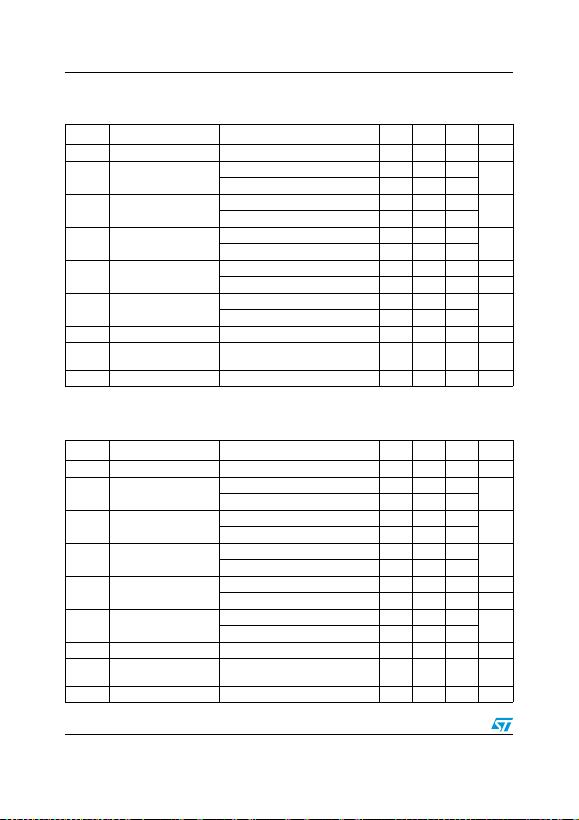Electrical characteristics L78LxxAB, L78LxxAC, L78LxxC
14/30 Doc ID 2145 Rev 20
TJ = 0 to 125 °C for L78L12AC, TJ = -40 to 125 °C for L78L12AB.
TJ = 0 to 125 °C for L78L15AC, TJ = -40 to 125 °C for L78L15AB.
Table 19. Electrical characteristics of L78L12AB and L78L12AC (VI = 19 V)
Symbol Parameter Test conditions Min. Typ. Max. Unit
VOOutput voltage TJ = 25 °C 11.5 12 12.5 V
VOOutput voltage IO = 1 to 40 mA, VI = 14.5 to 27 V 11.4 12.6 V
IO = 1 to 70 mA, VI = 19 V 11.4 12.6
ΔVOLine regulation VI = 14.5 to 27 V, TJ = 25 °C 250 mV
VI = 16 to 27 V, TJ = 25 °C 200
ΔVOLoad regulation IO = 1 to 100 mA, TJ = 25 °C 100 mV
IO = 1 to 40 mA, TJ = 25 °C 50
IdQuiescent current TJ = 25 °C 6.5 mA
TJ = 125 °C 6 mA
ΔIdQuiescent current change IO = 1 to 40 mA 0.1 mA
VI = 16 to 27 V 1.5
eN Output noise voltage B =10 Hz to 100 kHz, TJ = 25 °C 80 µV
SVR Supply voltage rejection VI = 15 to 25 V, f = 120 Hz
IO = 40 mA, TJ = 25 °C 37 42 dB
VdDropout voltage 1.7 V
Table 20. Electrical characteristics of L78L15AB and L78L15AC (VI = 23 V)
Symbol Parameter Test conditions Min. Typ. Max. Unit
VOOutput voltage TJ = 25 °C 14.4 15 15.6 V
VOOutput voltage IO = 1 to 40 mA, VI = 17.5 to 30 V 14.25 15.75 V
IO = 1 to 70 mA, VI = 23 V 14.25 15.75
ΔVOLine regulation VI = 17.5 to 30 V, TJ = 25 °C 300 mV
VI = 20 to 30 V, TJ = 25 °C 250
ΔVOLoad regulation IO = 1 to 100 mA, TJ = 25 °C 150 mV
IO = 1 to 40 mA, TJ = 25 °C 75
IdQuiescent current TJ = 25 °C 6.5 mA
TJ = 125 °C 6 mA
ΔIdQuiescent current change IO = 1 to 40 mA 0.1 mA
VI = 20 to 30 V 1.5
eN Output noise voltage B =10 Hz to 100 kHz, TJ = 25 °C 90 µV
SVR Supply voltage rejection VI = 18.5 to 28.5 V, f = 120 Hz
IO = 40 mA, TJ = 25 °C 34 39 dB
VdDropout voltage 1.7 VL78LxxAB, L78LxxAC, L78LxxC Electrical characteristics
Doc ID 2145 Rev 20 15/30
TJ = 0 to 125 °C for L78L18AC, TJ = -40 to 125 °C for L78L18AB.
TJ = 0 to 125 °C for L78L24AC, TJ = -40 to 125 °C for L78L24AB.
Table 21. Electrical characteristics of L78L18AC (VI = 27 V)
Symbol Parameter Test conditions Min. Typ. Max. Unit
VOOutput voltage TJ = 25 °C 17.3 18 18.7 V
VOOutput voltage IO = 1 to 40 mA, VI = 22 to 33 V 17.1 18.9 V
IO = 1 to 70 mA, VI = 27 V 17.1 18.9
ΔVOLine regulation VI = 22 to 33 V, TJ = 25 °C 320 mV
VI = 22 to 33 V, TJ = 25 °C 270
ΔVOLoad regulation IO = 1 to 100 mA, TJ = 25 °C 170 mV
IO = 1 to 40 mA, TJ = 25 °C 85
IdQuiescent current TJ = 25 °C 6.5 mA
TJ = 125 °C 6 mA
ΔIdQuiescent current change IO = 1 to 40 mA 0.1 mA
VI = 23 to 33 V 1.5
eN Output noise voltage B =10 Hz to 100 kHz, TJ = 25 °C 120 µV
SVR Supply voltage rejection VI = 23 to 33 V, f = 120 Hz
IO = 40 mA, TJ = 25 °C 33 38 dB
VdDropout voltage 1.7 V
Table 22. Electrical characteristics of L78L24AB and L78L24AC (VI = 33 V)
Symbol Parameter Test conditions Min. Typ. Max. Unit
VOOutput voltage TJ = 25 °C 23 24 25 V
VOOutput voltage IO = 1 to 40 mA, VI = 27 to 38 V 22.8 25.2 V
IO = 1 to 70 mA, VI = 33 V 22.8 25.2
ΔVOLine regulation VI = 27 to 38 V, TJ = 25 °C 350 mV
VI = 28 to 38 V, TJ = 25 °C 300
ΔVOLoad regulation IO = 1 to 100 mA, TJ = 25 °C 200 mV
IO = 1 to 40 mA, TJ = 25 °C 100
IdQuiescent current TJ = 25 °C 6.5 mA
TJ = 125 °C 6 mA
ΔIdQuiescent current change IO = 1 to 40 mA 0.1 mA
VI = 28 to 38 V 1.5
eN Output noise voltage B =10 Hz to 100 kHz, TJ = 25 °C 200 µV
SVR Supply voltage rejection VI = 29 to 33 V, f = 120 Hz
IO = 40 mA, TJ = 25 °C 31 37 dB
VdDropout voltage 1.7 V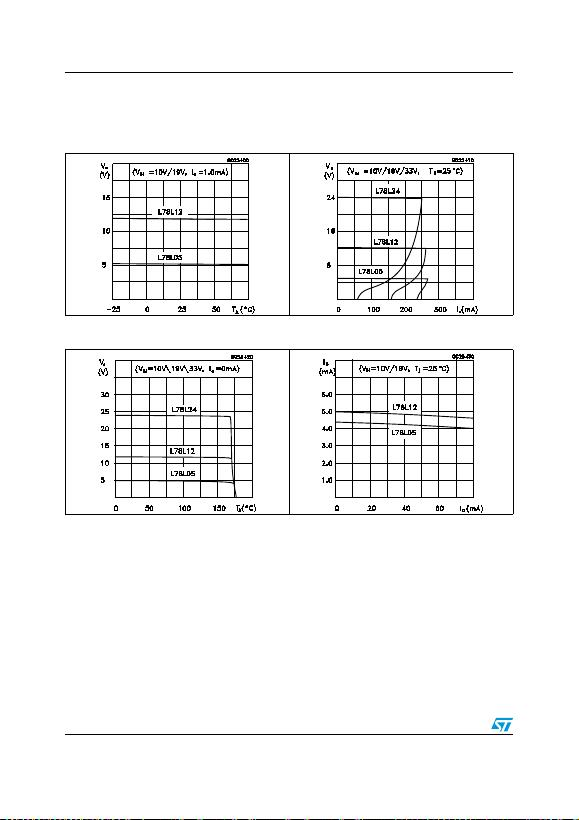Typical performance L78LxxAB, L78LxxAC, L78LxxC
16/30 Doc ID 2145 Rev 20
5 Typical performance
Figure 4. L78L05/12 output voltage vs.
ambient temperature